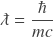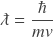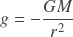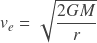# The Structure of Natural Formulas

The following table demonstrates the structure of natural formulas in which composite constants are replaced by fundamental Planck units:

### natural formula

Compton wavelengthde Broglie wavelengthmomentumphoton energyphoton energyelectron energygravitational accelerationgravitational forcegravitational energyorbital velocityescape velocity